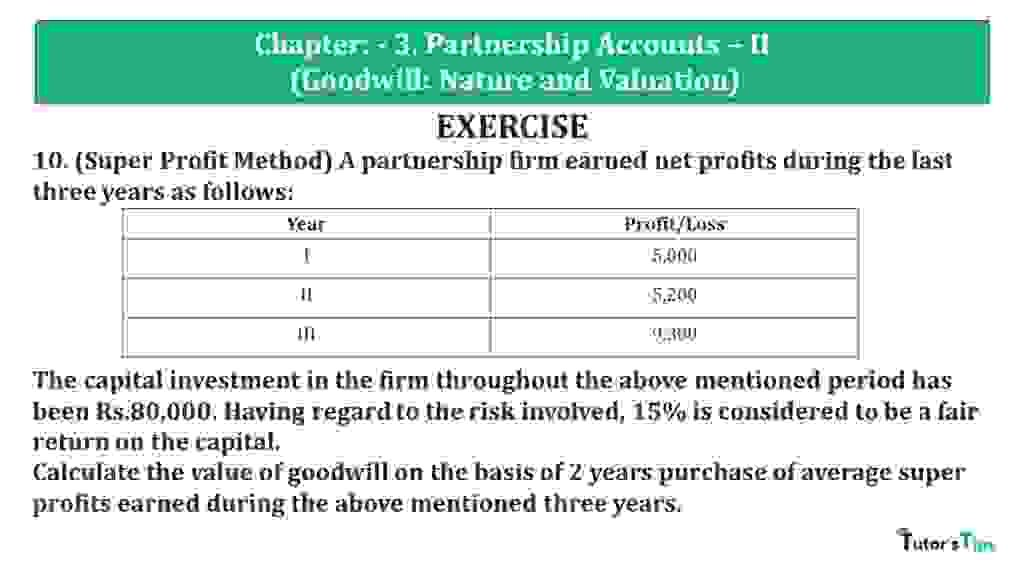# Question 10 Chapter 3 of +2 Part-1 – USHA Publication 12 Class Part – 1Question 10 Chapter 3 of +2-Part-1

10. (Super Profit Method) A partnership firm earned net profits during the last three years as follows:

 Year Profit/Loss I 5,000 II 5,200 III 9,300

The capital investment in the firm throughout the above mentioned period has been Rs.80,000. Having regard to the risk involved, 15% is considered to be a fair return on the capital.
Calculate the value of goodwill on the basis of 2 years purchase of average super profits earned during the above mentioned three years.

### The solution of Question 10 Chapter 3 of +2 Part-1: –

 Super Profit = Actual average Profit – Normal Profit Average Profit = Total Profit for past given years Number of years = 17,000 + 20,000 + 23,000 3 = 60,000 3 = 20,000

 Average Profit = Capital Employed X Normal Rate of Return 100 = 80,000 X 15 10 = 12,000

 Super Profit = 20,000 – 12,000 = 8,0000 Number of years’ purchase = 2 Goodwill = Super Profit X Number of years’ purchase Goodwill = 8,000 X 2 Goodwill = 16,000